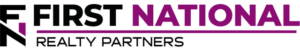# Understanding the Net Present Value Formula

### Key Takeaways

• Fundamentally, the Time Value of Money is a concept that states a dollar received today is worth more than a dollar received at some period of time in the future due to its ability to earn interest.
• Net Present Value is an investment metric that tells an investor if the property’s cash flows are achieving a target return, based on a proposed purchase price.

## Get Instant Access to All of FNRP’s Real Estate Deals

When an individual purchases a commercial real estate asset, they do so with the expectation that they will receive a return on their money.  In most cases, the return consists of some combination of income, which are periodic cash flows received at certain points in the investment holding period, and appreciation which is the difference between the initial price paid for the asset and the price that it is sold for.  But, trying to figure out the potential return and the price that must be paid to receive that return can be tricky.  One of the ways that real estate investors do this is to calculate an investment’s “Net Present Value” or “NPV.”

To understand what Net Present Value is and how it works, it is first important to understand two fundamental real estate concepts, the Time Value of Money and the Proforma.

## Time Value of Money and the Proforma

Fundamentally, the Time Value of Money is a concept that states a dollar received today is worth more than a dollar received at some period of time in the future due to its ability to earn interest.  On a larger scale, if a rational individual had the choice to receive an amount of money equal to \$1M today or \$1M in five years, they would choose to receive the money today because they could invest it and earn interest.  If they earn a positive return on investment, their initial outlay of \$1M should have a far higher valuation at the end of the same 5 year period.

As it relates to Net Present Value, the Time Value of Money concept works in reverse.  First, an investor forecasts a property’s expected cash flows into the future and then discounts them back using a “discount rate” that is roughly equal to their required return on the investment.  The result of this NPV analysis is the “Net Present Value” of the stream of cash flows.

To forecast a property’s cash flows, an investor creates a projection called a “proforma” which is simply a listing of a property’s expected cash flows and expenses, including debt service, for a desired holding period, usually 5-10 years.  The resulting net cash flow is what the discount rate is applied to when attempting to determine the Net Present Value.  At the highest level, a proforma looks like this (assuming the number of periods is 5 years):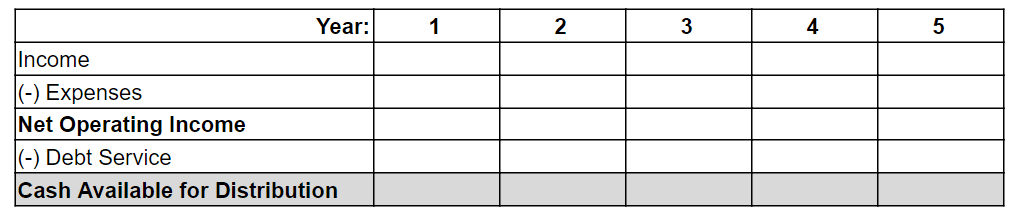The final line item in the proforma, Cash Available for Distribution, is the one that is used in the Net Present Value calculation.

## What is Net Present Value (NPV)?

Net Present Value is an investment metric that tells an investor if the property’s cash flows are achieving a target return, based on a proposed purchase price.  Put another way, it helps to determine the potential profitability of the investment, based on an assumed purchase price.  In addition, it also specifies the adjustment needed to reach the target return, assuming all other factors remain the same.  Mathematically, the NPV formula can be complicated.  It looks like this: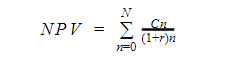Translated, this equation just means that a property’s Net Present Value (“NPV”) is equal to the sum of cash flows (C), for each period (n), discounted using the investor’s required rate of return (r). Fortunately, all major spreadsheet programs (Google Sheets and MS Excel) contain an “NPV” function so an investor does not have to manually calculate it.

The result of the NPV calculation can be one of three things:

• Positive Net Present Value (Positive NPV):  If the result of the calculation is a positive number, it means that the proposed purchase price is less than the asset is worth.  A higher NPV indicates a higher level of potential profitability.
• Negative Net Present Value (Negative NPV):  If the result of the calculation is a negative number, it means that the proposed purchase price is more than what the asset is worth.
• Zero:  If the result of the calculation is zero, it means that the investor is paying exactly what the asset is worth.  It should be noted that a result of zero also means that the discount rate is equal to the property’s internal rate of return or “IRR.”

The major factor in whether or not NPV is positive or negative is the choice of the “discount” rate.

## What is the Discount Rate?

In the equation, the Discount Rate is the rate of return used to discount future cash flows back to the present time.  The higher the rate, the more the cash flows will be discounted.  But, the rate chosen can vary from one investor to another and it represents the required rate of return on the investment.  So, if the property is deemed to have a higher degree of risk, like a ground up development project, an investor may demand a higher rate of return than if the investment is perceived to have a lower level of risk.

So, the short answer is that the choice of a discount rate is unique to each investor.  However, for most projects it can range from 5% – 12%.

## NPV – An Example

To illustrate how NPV works, an example is helpful.  Assume that a property is purchased for \$1M and that it produces annual cash flows for five years until it is sold at the end of year five for \$1.1M (the “future value”).  The \$1M purchase price (the initial investment) is expressed as a negative number because it represents a cash outflow, while the annual cash flows are expressed as positive numbers because they represent cash inflows.  The following tables displays a series of net cash flows and demonstrates the NPV calculation at varying discount rates: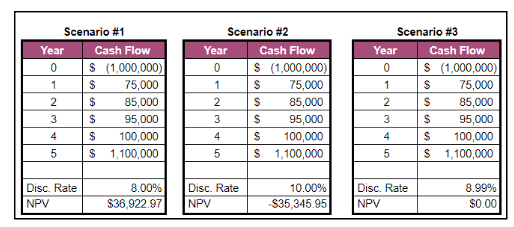All three scenarios have the same set of cash flows, but different discount rates are used.  In the first scenario, a discount rate of 8% is used and the resulting NPV is a positive number indicating that the purchase price of \$1M is below the asset’s value.  But, in the second scenario, if the investor demands a 10% rate of return, the resulting NPV is a negative number which means that the proposed purchase price of \$1M is too high.  Finally, in the last scenario, the NPV is \$0 with a discount rate of 8.99%, which means that the \$1M purchase price is exactly what the asset is worth for an investor who demands a return of 8.99%.  Again, the discount rate that sets the Net Present Value of all cash flows equal to \$0 is also known as the “Internal Rate of Return” or “IRR.”

The point is this, the Net Present Value of a series of cash flows can vary significantly based on the discount rate chosen.  Because the discount rate represents the rate of return that an investor wants to achieve, the perceived value of the property can vary from one investor to another.

## Interested in Learning More?

First National Realty Partners is one of the country’s leading private equity commercial real estate investment firms. With an intentional focus on finding world-class, multi-tenanted assets well below intrinsic value, we seek to create superior long-term, risk-adjusted returns for our investors while creating strong economic assets for the communities we invest in.

When underwriting our own investments, we use a variety of return metrics, including NPV, to determine the potential return on a given property.  If you would like to learn more about our investment opportunities, contact us at (800) 605-4966 or info@fnrealtypartners.com for more information.

## Get Access to Our CRE Deal Flow

Get instant access to all of our current and past commercial real estate deals.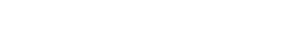## Subscribe Now

Get the latest news on real estate

## Get Instant Access to All of FNRP’s Real Estate Deals

Real Estate Investment Types

### September 30, 2022

Real Estate Investment Types

### September 29, 2022

Real Estate Investment Types

## Get More From FNRP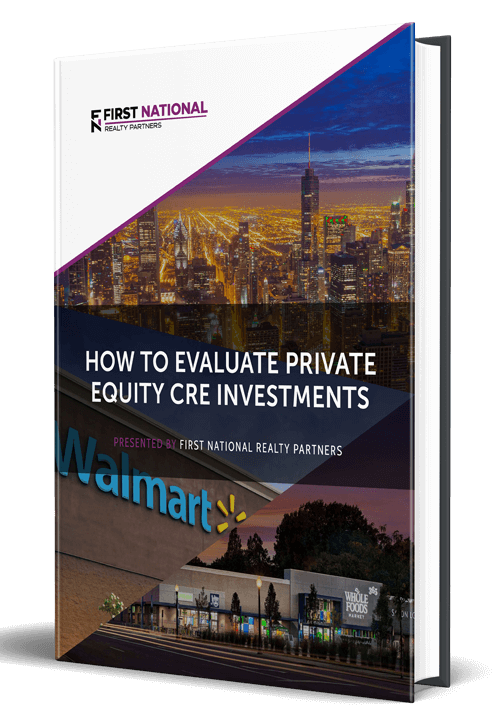## How to Evaluate Private Equity CRE Investments## The Ultimate Guide to Investing in Private Real Estate